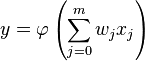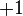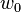# Artificial neuron

## Definition

An artificial neuron, also known as a semi-linear unit, Nv neuron, binary neuron, linear threshold function, or McCulloch–Pitts (MCP) neuron, is a function of the form:$y = \varphi\left(\sum_{j=0}^m w_j x_j\right)$

where$w_j$ are the weights on the neuron and$\varphi$ is the activation function. Artificial neurons form components of artificial neural networks: an artificial neural network is obtained by composing and combining artificial neurons (i.e., using the outputs of some neurons as inputs for other neurons).

Generally, in machine learning problems, the topology of the artificial neural network, as well as the choice of activation function for each neuron, are fixed in advance. The values of the weights are discovered using the training set by minimizing an appropriately chosen cost function.

### Bias term

Generally, the variable$x_0$ is always taken to be$+1$, and called the bias term. The weight$w_0$ is the bias weight.

### Purpose of the weights

The purpose of the weights is to combine the inputs in a way that extracts some information from all of them.

### Purpose of the activation function

The purpose of the activation function is to rescale in a manner that extracts the relevant valuable information from the linear combination. In general, the activation function tends to squish the domain down to a smaller subset. The idea is that the goal of the neuron is closer to a classification problem than a problem of finding an exact magnitude, so very large values should get squished down to the same value as intermediate values.

For instance, suppose a self-driving car is trying to determine whether a particular segment of the picture frame represents paved road or a sidewalk. The degree of certainty that the picture is of paved road can be described by a probability that can range from 0 to 1. We may compute this probability using a logistic regression problem: we combine a lot of different pieces of information about the picture frame to compute a real number describing the log-odds of it being paved road, then apply the logistic function to compute the probability. Here, the logistic function plays the role of the activation function.

A few other remarks:

• The logistic function is a fairly common choice of activation function, and the default artificial neural network architecture uses logistic functions at all artificial neurons, so we can view artificial neural networks as generalizations of logistic regression.
• Activation functions such as the logistic function, and most others that are typically chosen, have the property that for generally nice inputs, they are likely to simulate some form of almost-binary logic, and the artificial neural network can be viewed as a slight fuzzification of what is essentially a Boolean circuit.
• For an artificial neural network to have some power beyond a single artificial neuron, we must have a nonlinear activation function, because composing linear functions just gives us a linear function.